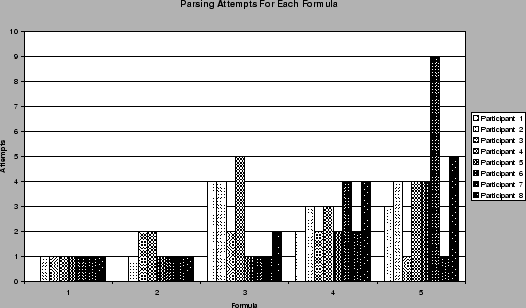Next: Evaluation of User Interface Up: Error Rates Previous: Misrecognised Characters

### Parsing Errors

Depending on the complexity of the formula the average number of rearranging steps before a formula was correctly parsed varied between zero (ideal), and eight.Figure 6.11 shows the number of parsing attempts for each formula by each participant in the user testing. If the number of parsing attempts is one, then the formula parsed correctly the first time and no rearranging steps were required. As the average number of parsing attempts across all the formulae was 2.3, the overall average number of rearranging steps was 1.3.

Steve Smithies
1999-11-13